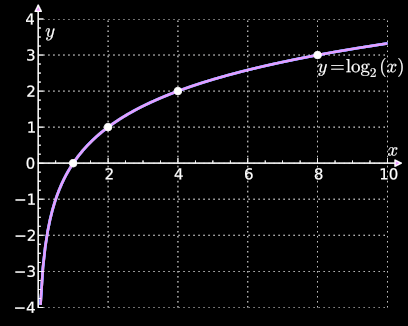# Logarithm tutorial overview

The following topics are covered in Arbital’s introductory guide to logarithms:

## 1. Definition of the logarithm

What is a logarithm function, anyway? You may have been told that it’s a function with a graph that looks like this:which is true, but not the whole story. This tutorial begins by asking: What is a logarithm?.

## 2. Log as length

Logarithms measure how long a number is, for a specific notion of “length” where fractional lengths are allowed. I don’t know what $$\log_{10}(\text{2,310,426})$$ is, but I can immediately tell you that it’s between 6 and 7, because it takes 7 digits to write 2,310,426:

$$\underbrace{\text{2,310,426}}_\text{7 digits}$$

Why is it $$\log_{10}$$ that measures length? What does it mean for a measure of “length” to be fractional? Why do logarithms say that it is 316 (rather than 500) that is 2.5 digits long? These questions and others are answered in Log as generalized length.

## 3. Logs measure data

Logarithms measure how hard it is to represent messages using physical objects in the world. Let’s say that we’re magicians, and I want to tell you what card I’m thinking of by using sleight-of-hand to arrange some dice. How many 6-sided dice do I need to set (without the audience noticing) to send you a message that tells you which card I’m thinking of, assuming there are 52 possibilities? The lower bound is $$\log_6(52).$$ Why? The answer reveals a link between logarithms and the “data capacity” of physical objects.

This idea is explored across three pages: Exchange rates between digits, Fractional digits, and Log as the change in the cost of communicating.

## 4. The characteristic of the logarithm

Any time you see a function $$f$$ such that $$f(x \cdot y) = f(x) + f(y)$$ for all $$x, y \in$$ $$\mathbb R^+$$, $$f$$ is a logarithm — more or less. The characteristic of the logarithm describes the main idea, and the optional Properties of the logarithm page takes you through the proofs and discusses some technicalities.

## 5. There is only one logarithm

While there are many logarithm functions (one for each positive number except 1), there is a sense in which they’re all doing exactly the same thing: Tapping into an intricate “logarithm lattice”.

## 6. Life in log-space

Empirically, logarithms have proved quite a useful tool for people and machines who have to do lots and lots of multiplications. Scientists and engineers used to use giant pre-computed tables of the logs of common numbers, and use those to make their calculations. Today, many modern learning algorithms (such as AlphaGo) manipulate the logs of probabilities instead of manipulating probabilities directly. Why? This tutorial concludes by exploring when and why it is easier to deal with the logarithms of things than it is to deal with the things themselves, on the page Life in logspace.

These six concepts are nowhere near all there is to say on the topic of logarithms. Logarithms have applications to many domains, including physics, computer science, calculus, number theory, and psychology. Logarithms have many interesting properties, such as nice derivatives, nice integrals, and interesting approximation algorithms. One of the bases of the logarithm, $$\log_e,$$ is “special.” The logarithm gets quite a bit more interesting when extended to the complex plane. Those are all more advanced topics, which aren’t covered in this tutorial. If you want to learn more about those sorts of topics, see Arbital’s advanced logarithm tutorial.

This guide focuses on building a solid intuition for what the logarithm does, and why it has its most important properties.

Parents: